# Lab 4B: Data Visualization (Hard)

1. Set the working directory

2. Read the policy CSV file into a data frame caled “policies”

### Problem 1: Visualizing One Categorical Variable

1. Create a frequency bar chart of Blood Type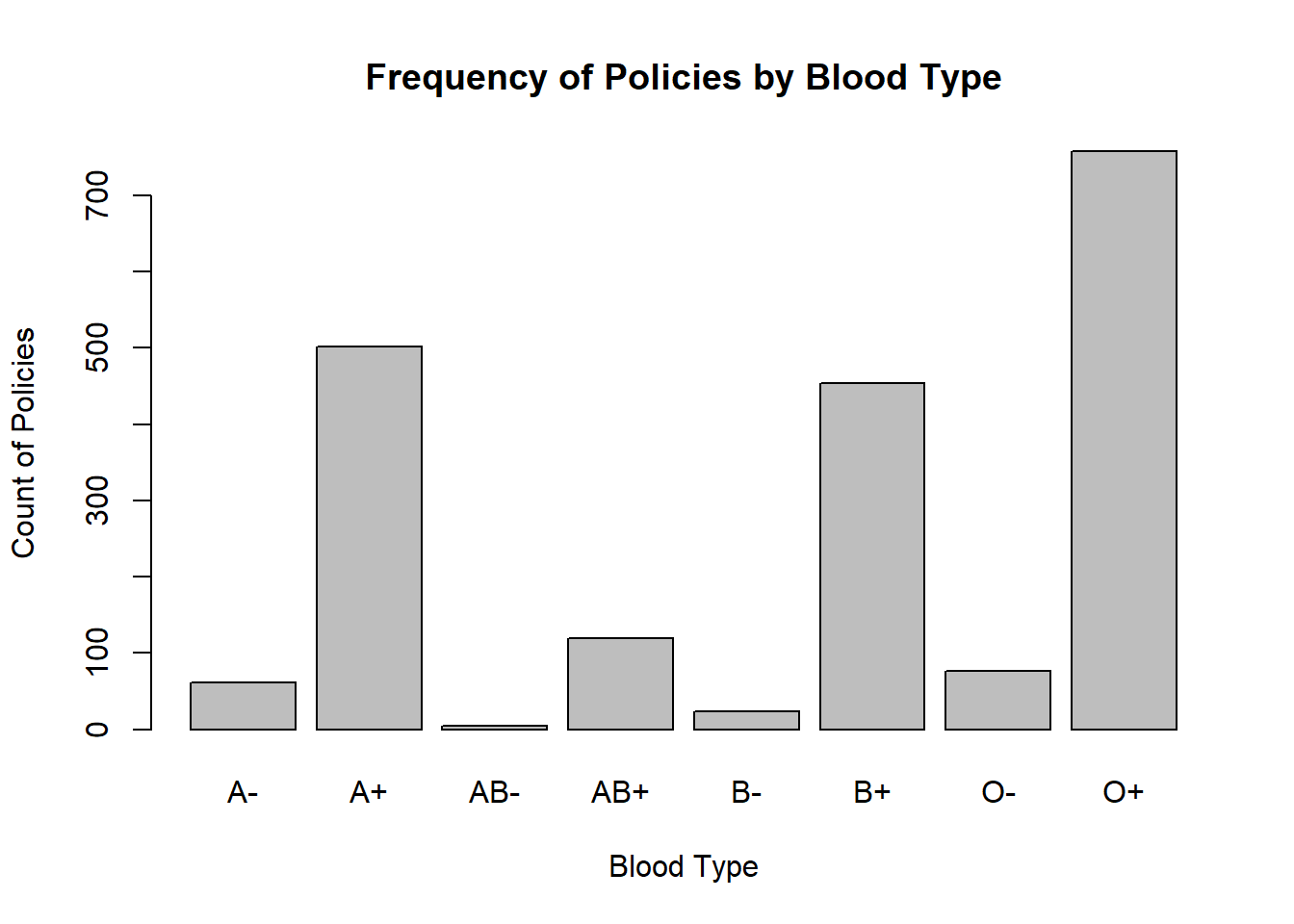Question: Which blood type is most common?

### Problem 2: Visualizing One Numeric Variable

1. Create a dot plot of height (Centimeters)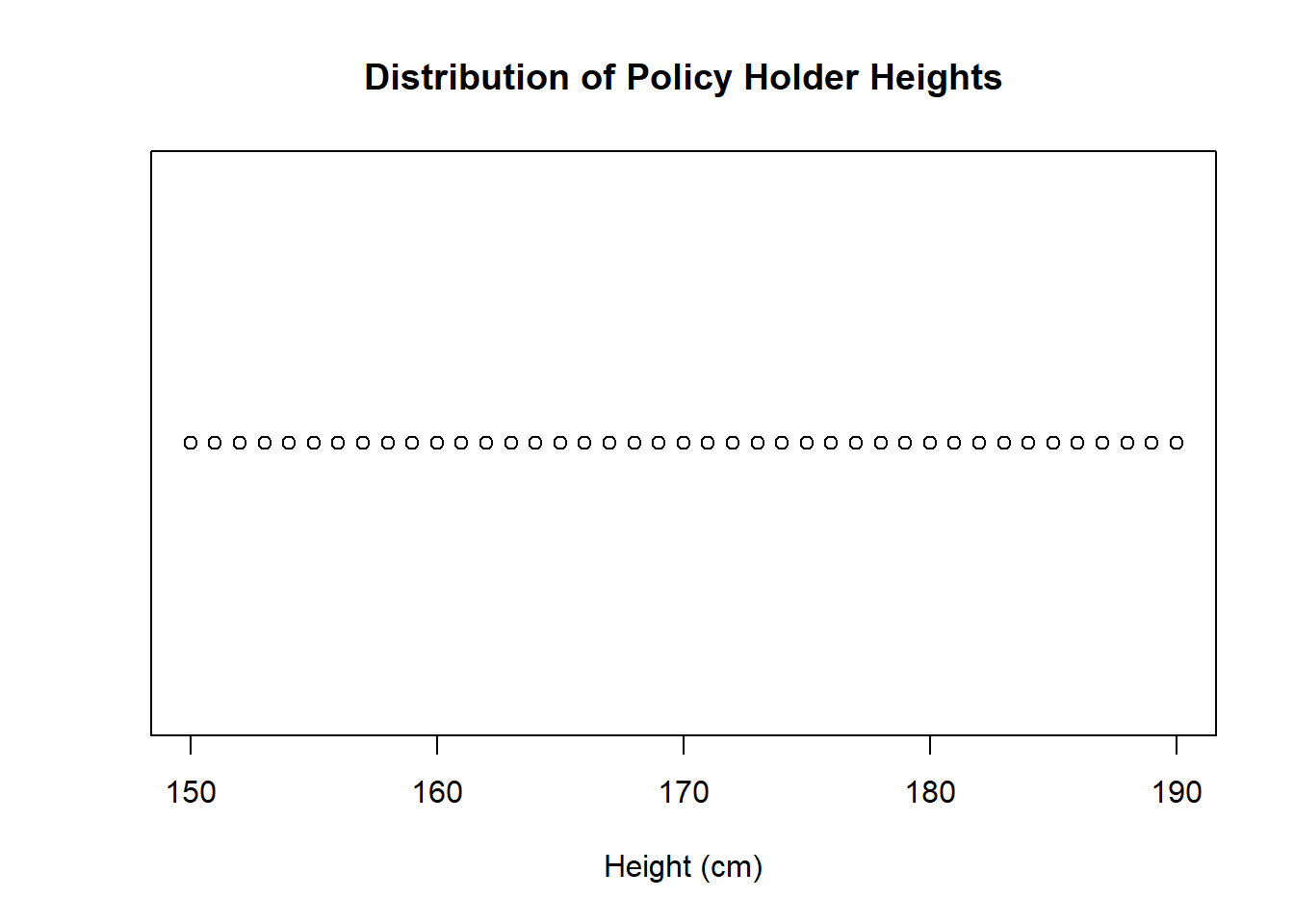2. Create a boxplot of height (Centimeters)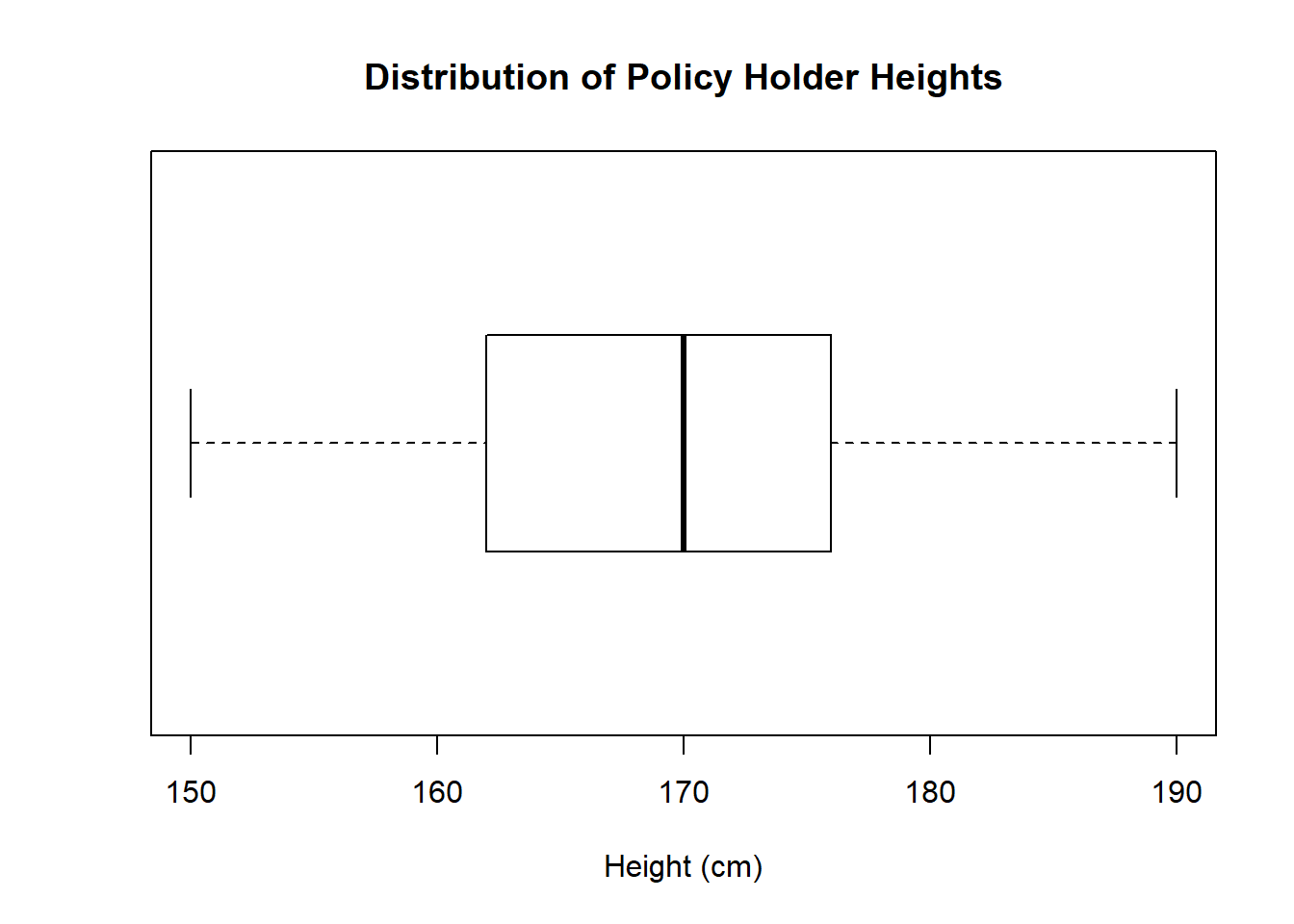3. Create a histogram of height (Centimeters)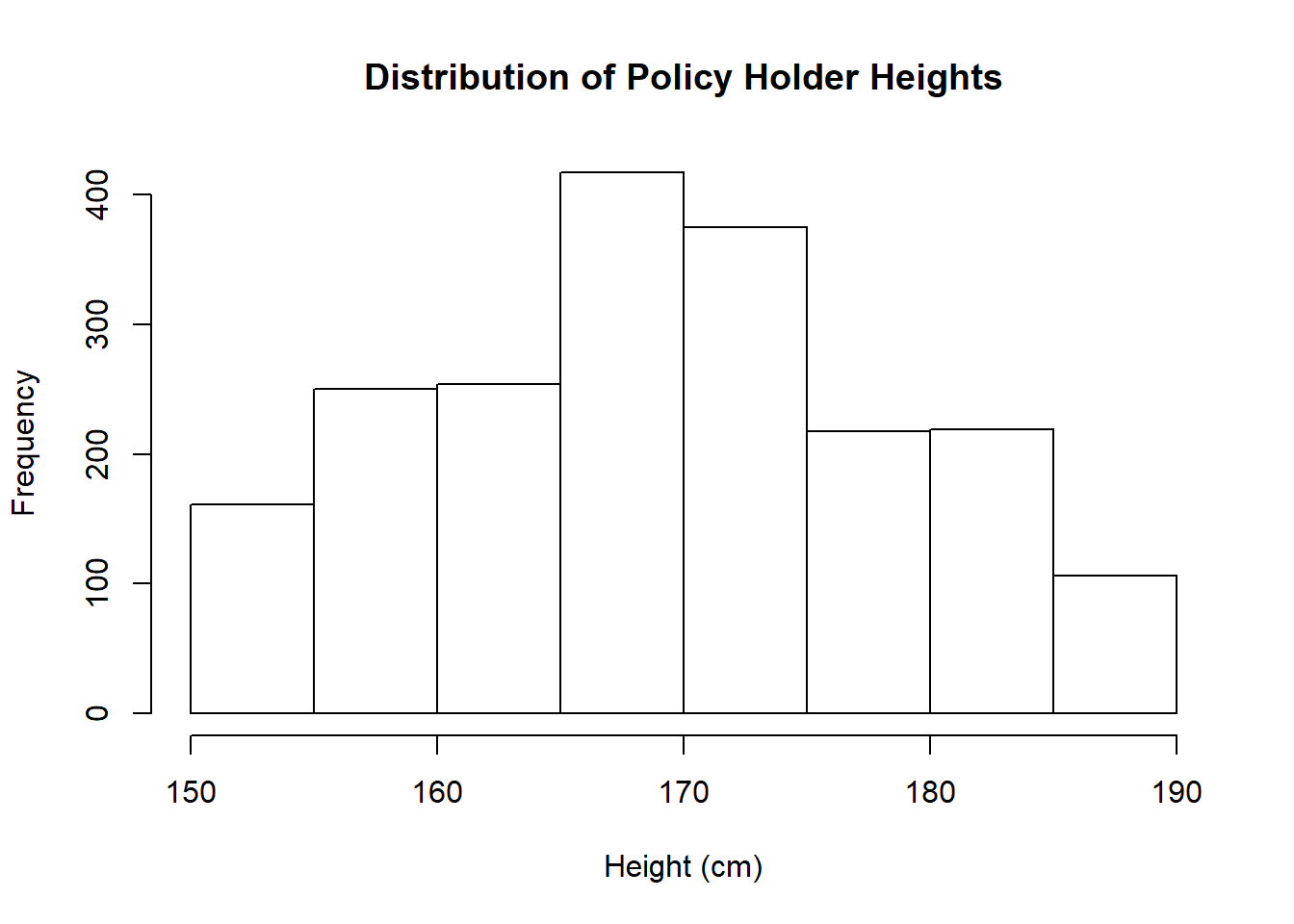4. Create a density plot of height (Centimeters)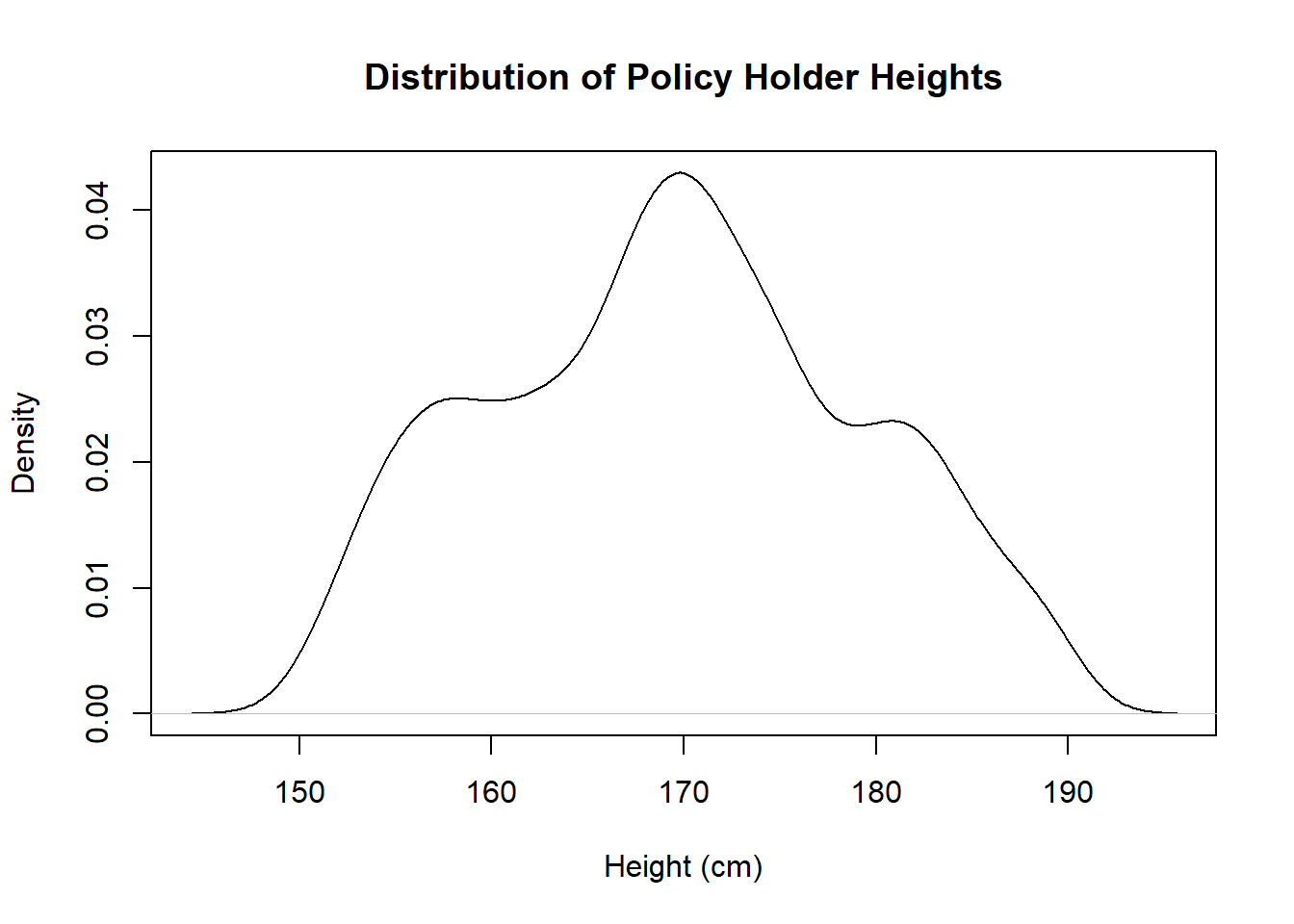Question: Is this distribution roughly symetric?

### Problem 3: Visualizing Two Categorical Variables

1. Create a spineplot of Blood Type and Gender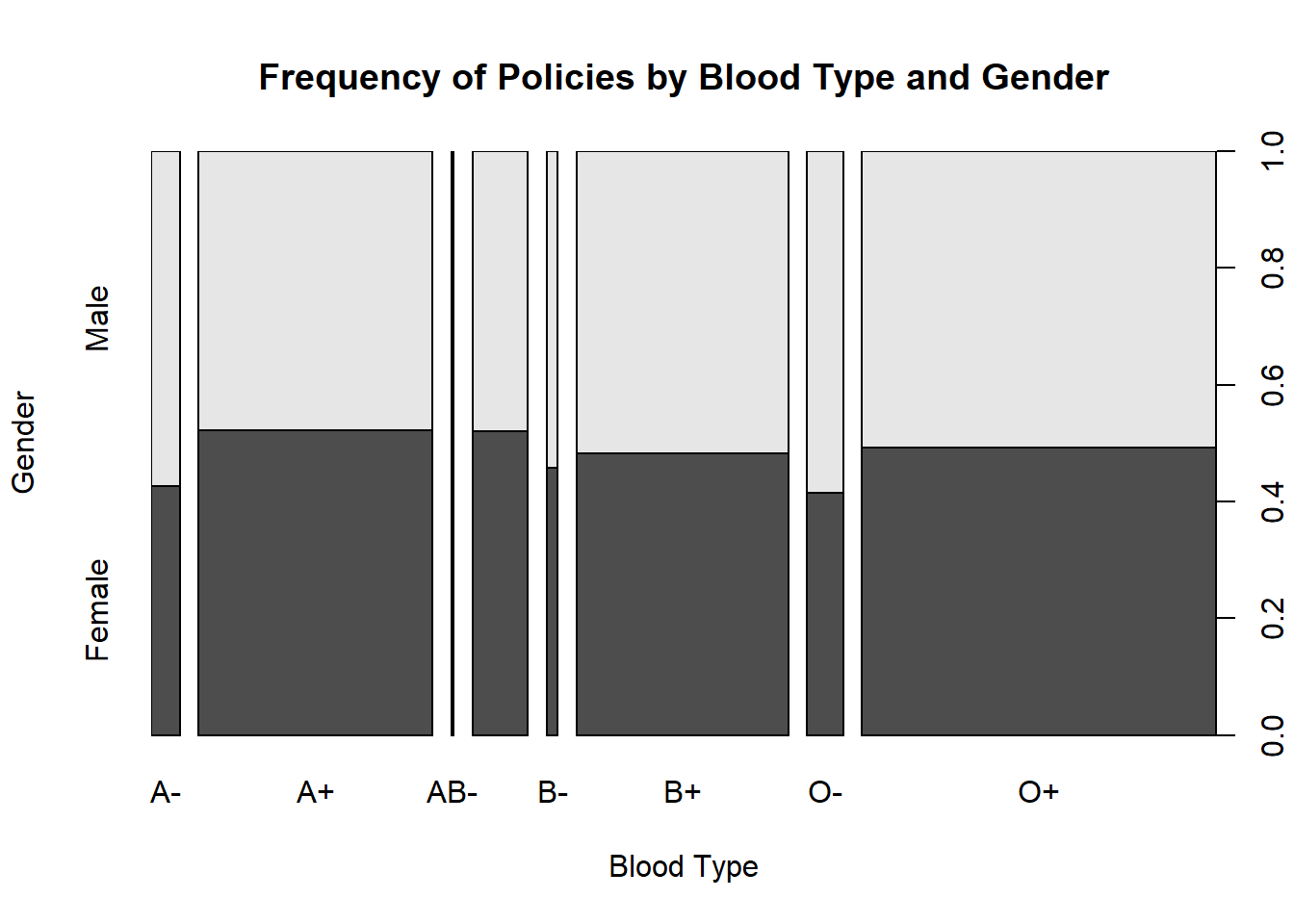2. Create a mosaic plot of blood type and gender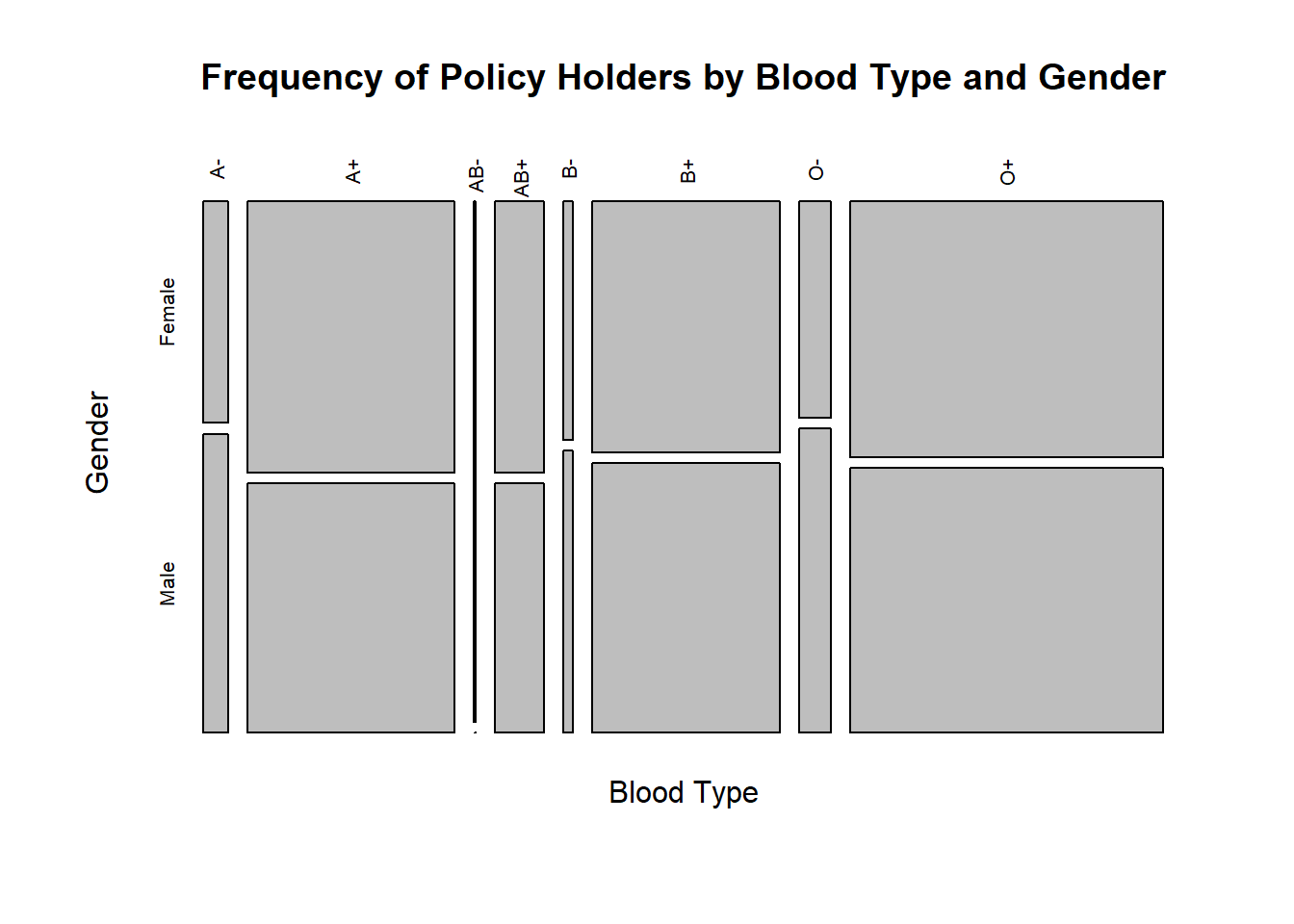Question: What appears to be the least common blood type for males?

### Problem 4: Visualizing Two Numeric Variables

1. Create a scatterplot of weight (Kilograms) and height (Centimeters)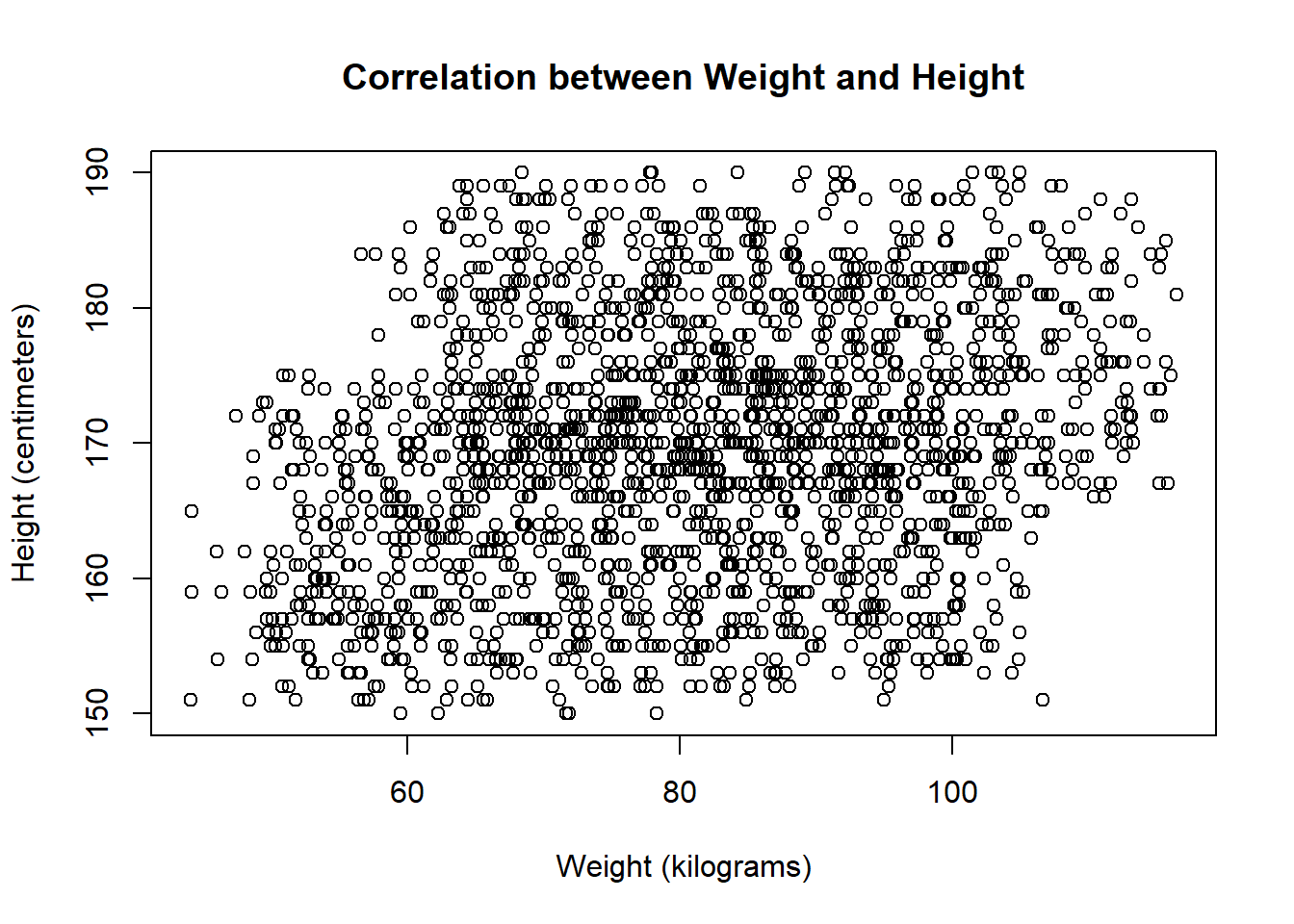2. Create a scatterplot of Rate and Age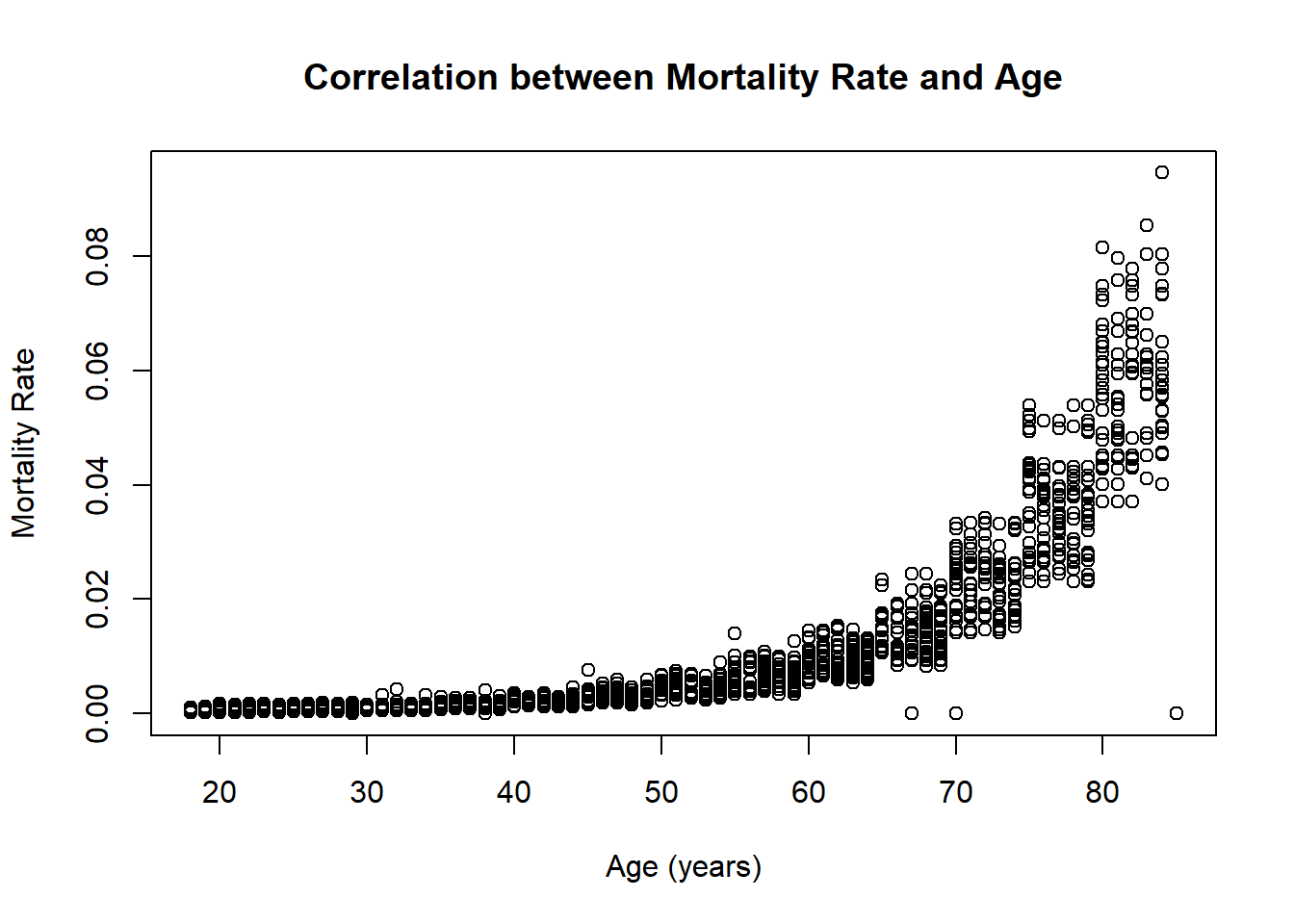Question: Does there appear to be any pattern in either of these plots?

### Problem 5: Visualize a Time Series

1. Plot a line graph of mortality rate by age
NOTE: We’re using Age as proxy for a time variable (i.e.Date of Birth)
NOTE: Create the array using tapply first, then set x to the names and y to the values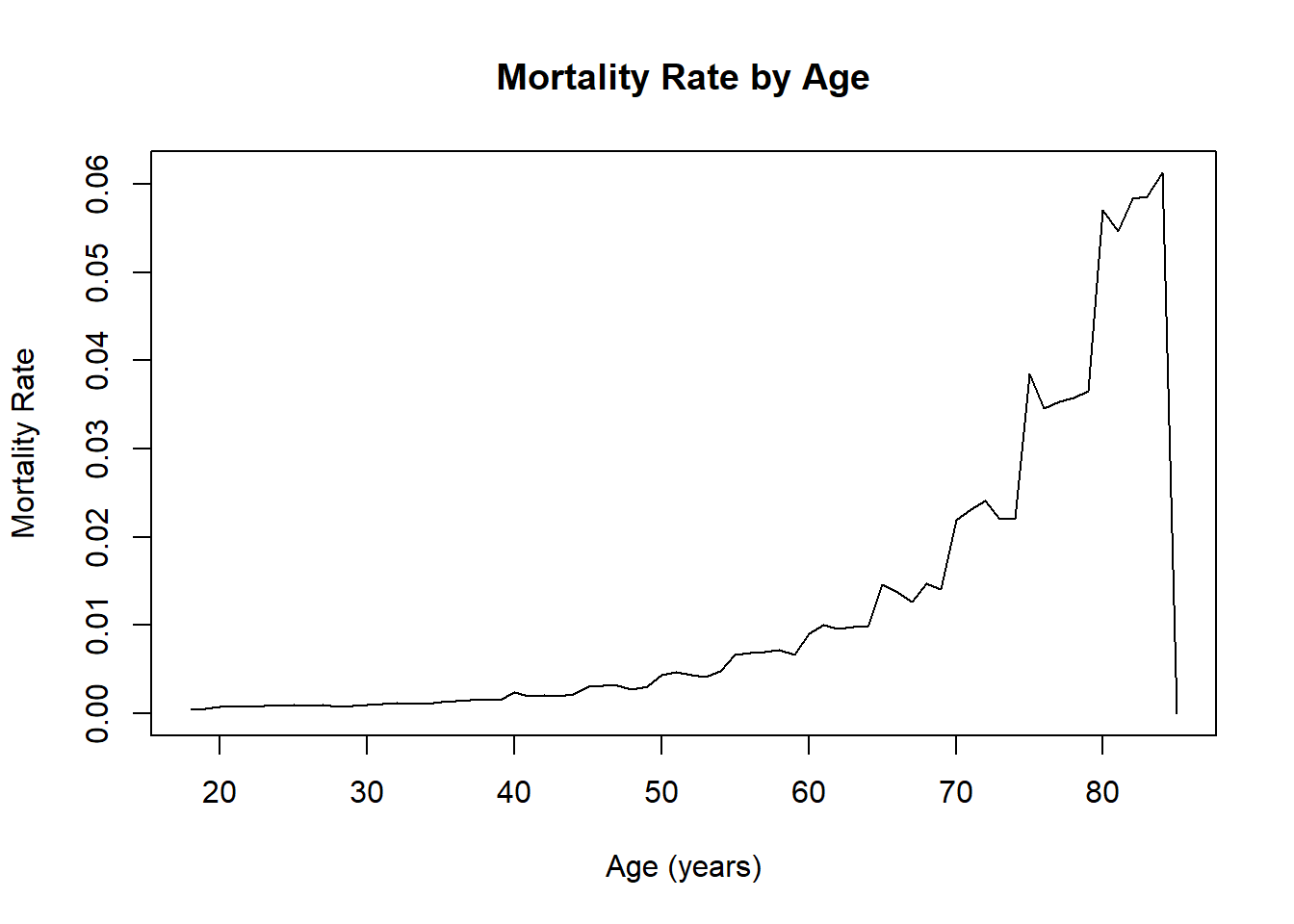Question: Why is there a steep decline at 85?

### Problem 6: Visualizing a Numeric Variable Grouped By a Categorical Variable

1. Create a bar graph of Rate by Gender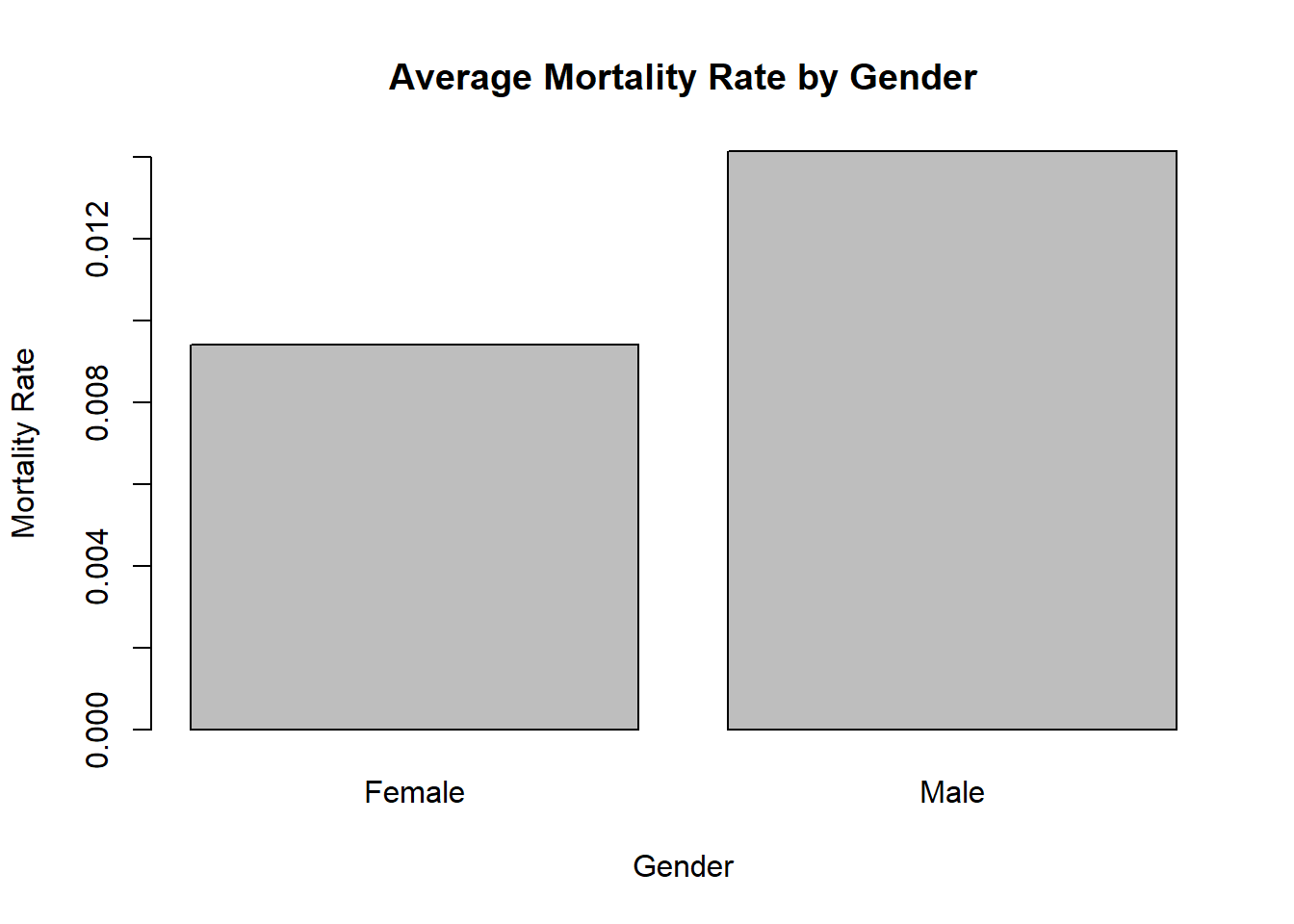2. Create a bar graph of average Height by Blood Type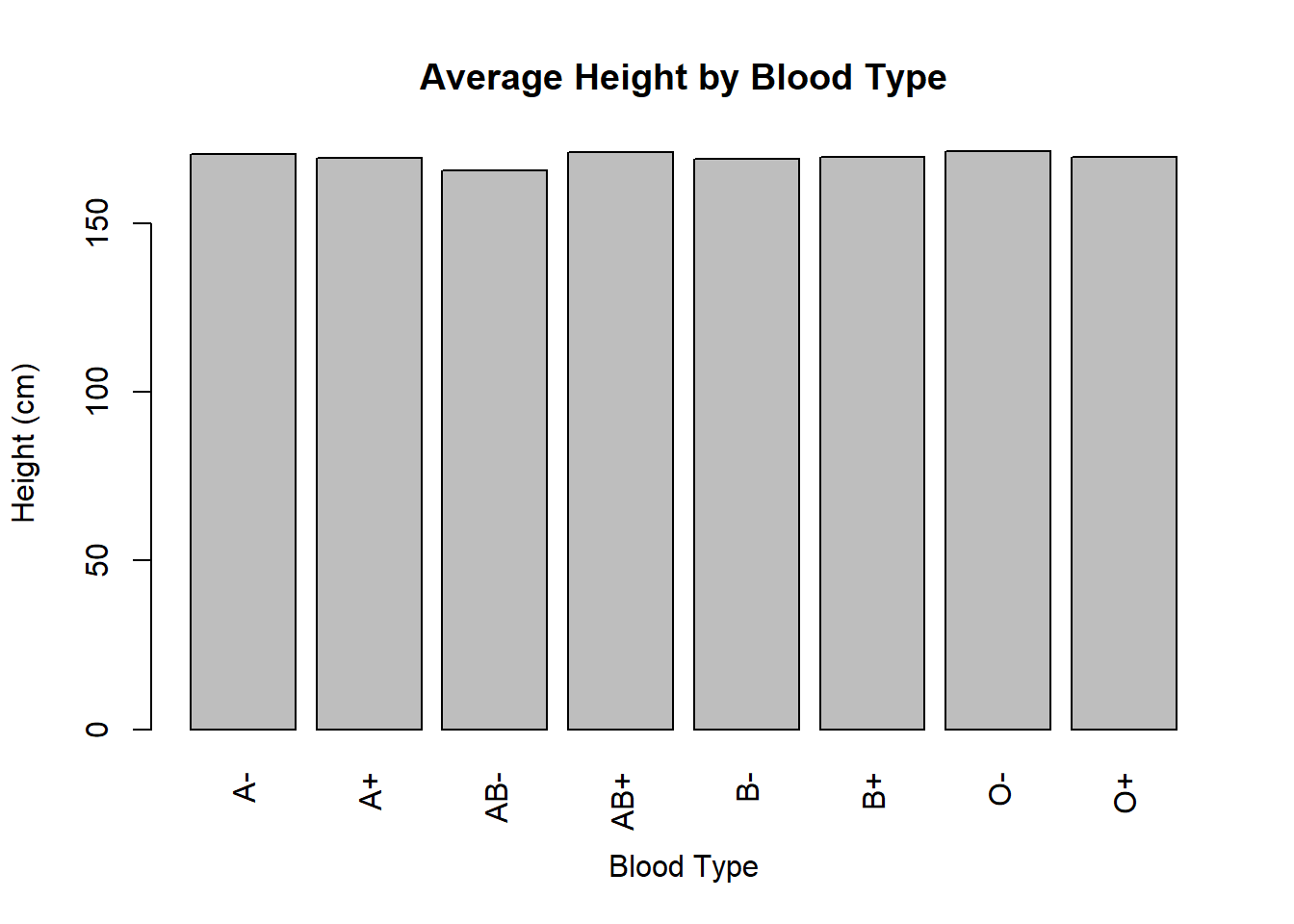Question: How are blood type and height related?

### Problem 7: Visualizing Many Variables

1. Create a scatterplot matrix of Age, Kilograms, Centimeters, and Rate